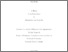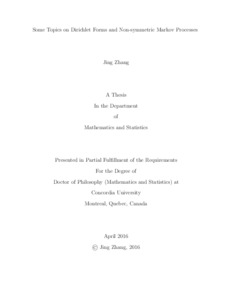Title:

# Some Topics on Dirichlet Forms and Non-symmetric Markov Processes

Zhang, Jing (2016) Some Topics on Dirichlet Forms and Non-symmetric Markov Processes. PhD thesis, Concordia University.Preview Text (application/pdf) Zhang_PhD_S2016.pdf - Accepted Version 1MB

## Abstract

In this thesis, we discuss three topics on Dirichlet forms and non-symmetric Markov processes.

First, we explore the analytic structure of non-symmetric Markov processes. Let U be an open set of \mathbf{R}^n, m a positive Radon measure on U, and (P_t)_{t>0} a strongly continuous contraction sub-Markovian semigroup on L^2(U;m). We give an explicit Levy-Khintchine type representation of the generator A of (P_t)_{t>0}. If (P_t)_{t>0} is an analytic semigroup, we give an explicit characterization of the semi-Dirichlet form {\cal E} associated with (P_t)_{t>0}.

Second, we consider the Dirichlet boundary value problem
for a general class of second order non-symmetric elliptic operators L with singular coefficients. We show that there exists a unique, bounded continuous solution by using the theory of Dirichlet forms and heat kernel estimates. Also, we give a probabilistic representation of the non-symmetric
semigroup generated by L.

Finally, we present new results on Hunt's hypothesis (H) for Levy processes. These include a comparison result on Levy processes which implies that big jumps have no effect on the validity of (H), a new necessary and sufficient condition for (H), and an extended Kanda-Forst-Rao theorem.

Divisions: Concordia University > Faculty of Arts and Science > Mathematics and Statistics Thesis (PhD) Zhang, Jing Concordia University Ph. D. Mathematics 1 April 2016 Sun, Wei 980977 JING ZHANG 16 Jun 2016 15:58 18 Jan 2018 17:52
All items in Spectrum are protected by copyright, with all rights reserved. The use of items is governed by Spectrum's terms of access.

Repository Staff Only: item control pageResearch related to the current document (at the CORE website)
Back to top